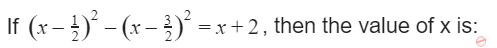October 12, 2023
October 12, 2023
October 12, 2023
###### P-NP
October 12, 2023
 Question 8A 2 B 4 C 8 D 6
Question 8 Explanation:

Expand the given equation:

x2+1/4 – x –x2 -9/4 +3x = x+2

=> -2 + 2x = x + 2

X = 4

Question 8 Explanation:

Expand the given equation:

x2+1/4 – x –x2 -9/4 +3x = x+2

=> -2 + 2x = x + 2

X = 4